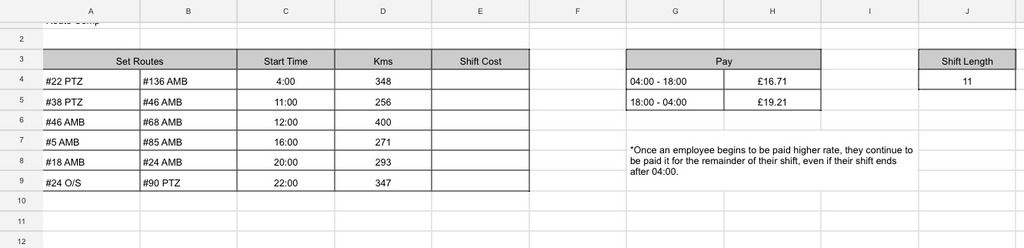Thread: Shift Pay CalculationsThanks: 0Likes: 0

1.Shift Pay Calculations

Need some help please; I'm working on a cost comparison and need some help with a sum that involve shift length, different rates depending on the time of day and the start time of the shift.E.g.
- If an eployee starts at 04:00 set in column C, I would like the sum to pay the correct "04:00-18:00" rate of pay, for the hours in the "shift length" cell. (11 hours at H4)

- If an employee starts at 12:00, I would like the sum pay them the correct proportion of rates. (11 hours shift as per J4, 6 hours at the rate in H4 and 5 hours at H5)

- If an employee starts at 22:00, I would like the sum to pay them the rate for the shift length in J4, however once an employee is paid a higher rate it cannot drop even if they work after 04:00. (11 hours at H5 regardless that they will be working after 04:00)

Thank you very much for any assistance.Reply With Quote

2.Re: Shift Pay Calculations

Try this in E4 and copy downward.

=IF(OR(HOUR(C4)>=18, HOUR(C4)< 4), \$H\$5*\$J\$4,
IF(AND(HOUR(C4)>=4, HOUR(C4)+\$J\$4<=18), \$H\$4*\$J\$4,
\$H\$4*(18-HOUR(C4)) + \$H\$5*(\$J\$4+HOUR(C4)-18)))Reply With Quote

3.Re: Shift Pay Calculations

Thank you very much, Ill try tomorrow. 👍Reply With Quote

User Tag List

Tags for this Thread

hours, pay, rate, shift, sumPosting Permissions

• You may not post new threads
• You may not post replies
• You may not post attachments
• You may not edit your posts
•